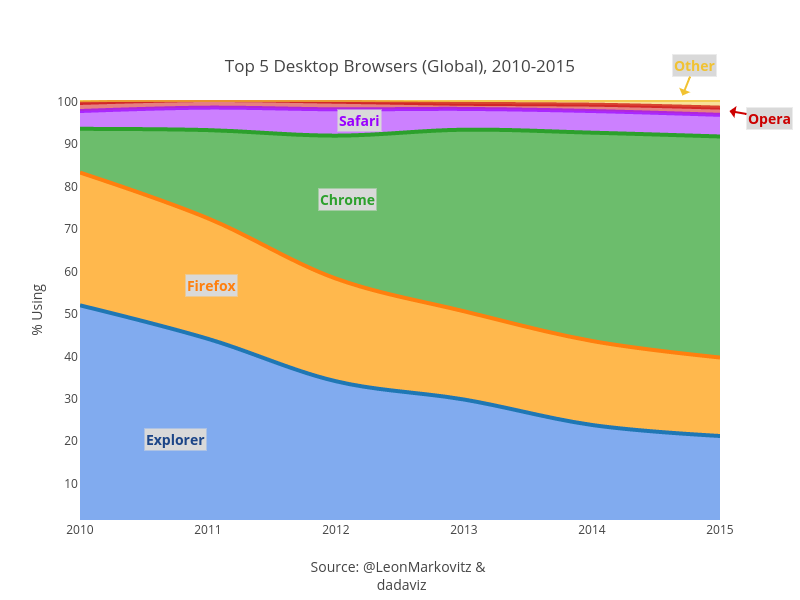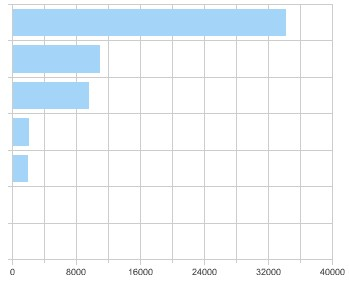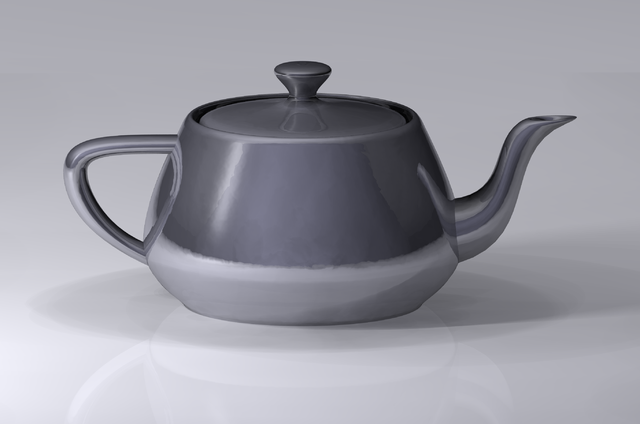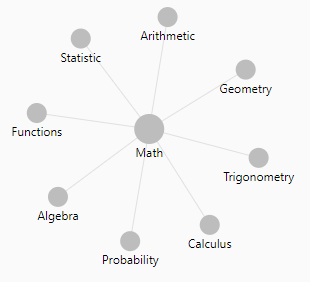# Data Visualization - ( Chart | Graph | Graphic)

A chart, also called a graph (in mathematics for instance), is a graphical representation of data, in which the data is represented by symbols, such as:

It's derived from the latin term grapho (to write)

## Representation

A chart can represent:

## Documentation / Reference

Discover MoreD3 - SVG module

The svg module provides various shapes suitable for charting. The mbostock/d3/wiki/SVG-Shapesarc function, builds elliptical arcs as for pie and donut charts by mapping arbitrary data to paths; typically...Data Visualisation - Area Chart

An area chart shows the trend of the contribution of: each value over time or by category. It is basically a line graph for which the regions between lines are filled in. Regions stack, adding...Data Visualization - Bar Chart

Bar graphs plots: a categorical variable associated with quantities (number of case, sum of) histograms continuous variable associated with its bins They show: quantities as bar lengths...Data Visualization - Gauge

A gauge shows a single data value. Due to its compact size, a gauge is often more effective than a graph for displaying a single data value. Gauges identify problems in data. A gauge usually plots...Data Visualization Foundation

The Utah Teapot. Data visualization is the process of mapping quantitative data to visuals (shapes, color, position, etc) to create a graph made of geometric object. Information visualization: ...Data Viz - Diagram

A diagram is a visual representation of a graph. It's a chart with arrows between visuals. A flow chart is a diagram that represents a directed graph SeeData Vizualization - Encoding / Decoding

Graphical perception: the visual decoding of information encoded on graphs. ie when people extract quantitative information from graphs. Data visualization encode the quantitative information in visual...Geometry (Spatial, Linear Algebra)

A geometry is the geometric representation of an object in some coordinate system. A graphic is the combination of this objects in this coordinate system. Same as ! ...Geometry - Curve (Parabola|Parabolic|Arche)

Parabola's or Parabolic arcs are simply curves or arches. A parabolic arc is a section of a parabola. A parabola is the curve graph of a quadratic function. parabola ...Mathematics - Quadratic (function|polynomial of degree 2)

In mathematics, a quadratic function, a quadratic polynomial, a polynomial of degree 2, or simply a quadratic, is a polynomial function in one or more variables in which the highest-degree...